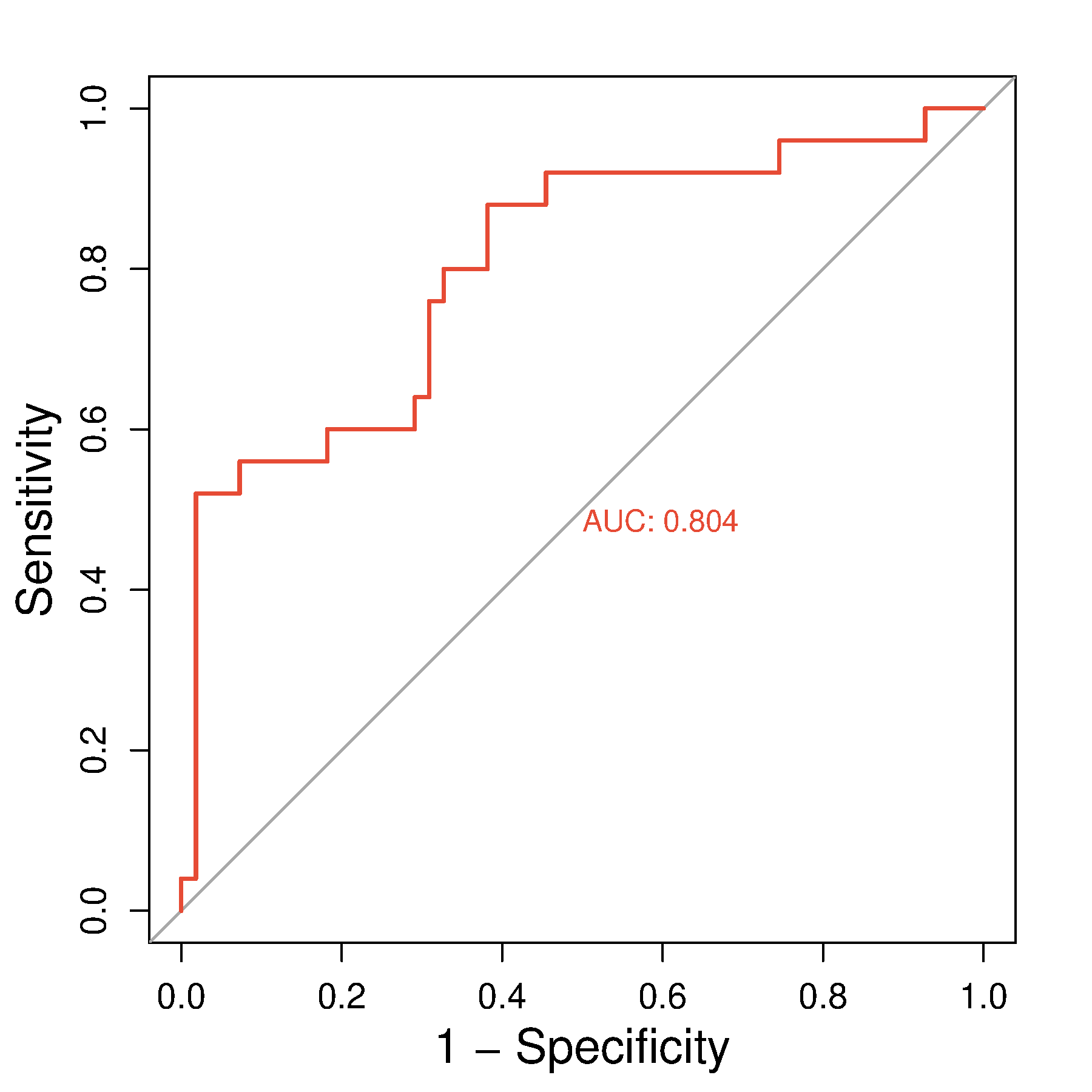Note: input data format must match the example on the right, tab-seperated
1, data from excel, copy and paste data into the input frame
2, data from txt, must tab-seperated, copy and paste data into the input frame
3, use point as decimal separator, not comma. e.g. 3.14, not 3,14 as pi

Required
input data (with header line, at most 10 curves)

Optional
figure size
figure width:
figure height:

fontsize
axis label fontsize:
axis ticks fontsize :

Colors
color 1: , color 2:
color 3: , color 4:
color 5: , color 6:
color 7: , color 8:
color 9: , color 10:

Smooth

X label

Percentage

Fontfamily

### ROC curve

Introduction
Receiver Operating Characteristic (ROC) curve. The X axis is false positive rate (FPR), Y axis is true positive rate (TPR). For certain classifier, we can get a (TPR, FPR) point pair based on the performance on test sets. Then, by adjusting the thresholds, we can get a curve from (0,0) to (1,1), this is the ROC curve of this classifier. Generally, this curve will be bellow the (0,0) (1,1) diagonal line. Because this diagonal line represents a random classifier. If what your curves are on top of this diagonal line, just exchange your classifier outputs. Although ROC curve is straightforward in representing the performance of the classifier, but we want a value to represent good or bad, that is Area Under roc Curve (AUC). Generally, AUC values are between 0.5-1.0, larger AUC represent better classification performance.
Input data instructions
The first column is sample, the second column is class (binary), the other columns are expression values of certain gene. plot with pROC package.
Examples from paperspROC: an open-source package for R and S+ to analyze and compare ROC curves. Fig2
 Input Example Output1) How to plot?
1, Prepare data
2, Open with excel, and change into the same format as the example
3, Copy and paste data into the input frame
4, Select parameters